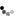about the topic of cost of captal

20 June 2010 sir,please suggest me how to work out for finding specific cost of capital for debenture and prefrence share when we have to find out:
weighted cost of capial as per book value
weighted cost of capital as per market value

20 June 2010 U should follow the following steps:-
1) First u calculate the specific cost of debenture and preference share by applying the respective formula
2)U shall take the weight of book value of deb and pref share or eqity sh if any,if requirement is book value but where the requirement is market value u take weight of mkt value of respective capital
3)MUltiply the weight by specific cost and u would get the overall cost of capital
4)Specific cost is same in both the calculation

You need to be the querist or approved CAclub expert to take part in this query .

Similar Resolved Queries